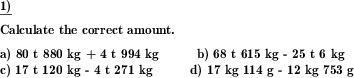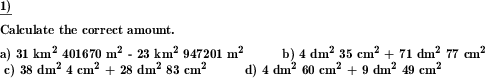Custom math worksheets at your fingertips# Details for problem "Units of measurement add and subtract"

Quickname: 7543

Elementary School, Primary School, Middle School.

## Summary

Add and subtract units of measurement

## Examples## Description

Units of measurement have to be added and subtracted for different physical quantities.

The physical quantities available are

• Money (Euro, Cent)
• Money (dollars, cents)
• Length (metric units)
• Volume (metric units, litres)
• Volume (metric units, dm^3)
• Time (hms)
• Time (dhms)
• Weight (metric units)

The number of problems is selectable. The number of units that may be covered in a single problem can be limited, this means when two units are selected, km dm and m will not be all part of a single specification, but only dm and m, m and dm, dm and cm etc.

The number of summands can be selected. Problems may be presented as addition or subtraction problems or a mix of both.

Download free printable worksheets for this math problem here. The worksheet contains the problems only, the solution sheet includes the answers. Just click on the respective link.

•Worksheet 1Solution sheet with answers
•Worksheet 2Solution sheet with answers
•Worksheet 3Solution sheet with answers

If you can not see the solution sheets for download, they may be filtered out by an ad blocker that you may have installed. If this is the case, please allow ads for this page and reload the page. The solution sheets will then reappear.

• Do these sample worksheets do not really fit?
• Do you need more math worksheets, with a different level of difficulty?
• Would you like to combine different problems on a worksheet and adjust them to your needs?
• As a teacher, you can put together your own worksheets using the automatically generated math problems provided.
With a free initial credit, you can start creating your own math worksheets in a few minutes.

You can try it for free! Register here, to create custom worksheets now!

## Customization options for this problem

Parameter
Possible values
Number of problems
1, 2, 3, 4, 5, 6, 7, 8, 9, 10
Physical quantity
Area (metric), Length (metric), Weight (metric), Time (dhms), Time (hms), Volume litres (metric), Volume dm2 (metric), Money (USD), Money (EUR)
Number of summands
2, 3, 4, 5, 6
Mixed, Plus, Minus
Max number of units
2, 3, 4, 5, 6
Include single unit values
Yes, No

## Similar problems

Remark
Description
Determine fraction, percentage of units of measurement
Identify fractions of area, price, weight, length given in different forms: 1/8, 20%, 0.3.
In the form of a pyramid
Fill in the blank boxes in the pyramid of units of measurement.